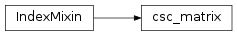# mvpa2.algorithms.searchlight_hyperalignment.csc_matrix¶class `mvpa2.algorithms.searchlight_hyperalignment.``csc_matrix`(arg1, shape=None, dtype=None, copy=False)

Compressed Sparse Column matrix

This can be instantiated in several ways:

csc_matrix(D)
with a dense matrix or rank-2 ndarray D
csc_matrix(S)
with another sparse matrix S (equivalent to S.tocsc())
csc_matrix((M, N), [dtype])
to construct an empty matrix with shape (M, N) dtype is optional, defaulting to dtype=’d’.
csc_matrix((data, (row_ind, col_ind)), [shape=(M, N)])
where `data`, `row_ind` and `col_ind` satisfy the relationship `a[row_ind[k], col_ind[k]] = data[k]`.
csc_matrix((data, indices, indptr), [shape=(M, N)])
is the standard CSC representation where the row indices for column i are stored in `indices[indptr[i]:indptr[i+1]]` and their corresponding values are stored in `data[indptr[i]:indptr[i+1]]`. If the shape parameter is not supplied, the matrix dimensions are inferred from the index arrays.

Notes

Sparse matrices can be used in arithmetic operations: they support addition, subtraction, multiplication, division, and matrix power.

• efficient arithmetic operations CSC + CSC, CSC * CSC, etc.
• efficient column slicing
• fast matrix vector products (CSR, BSR may be faster)
• slow row slicing operations (consider CSR)
• changes to the sparsity structure are expensive (consider LIL or DOK)

Examples

```>>> import numpy as np
>>> from scipy.sparse import csc_matrix
>>> csc_matrix((3, 4), dtype=np.int8).toarray()
array([[0, 0, 0, 0],
[0, 0, 0, 0],
[0, 0, 0, 0]], dtype=int8)
```
```>>> row = np.array([0, 2, 2, 0, 1, 2])
>>> col = np.array([0, 0, 1, 2, 2, 2])
>>> data = np.array([1, 2, 3, 4, 5, 6])
>>> csc_matrix((data, (row, col)), shape=(3, 3)).toarray()
array([[1, 0, 4],
[0, 0, 5],
[2, 3, 6]])
```
```>>> indptr = np.array([0, 2, 3, 6])
>>> indices = np.array([0, 2, 2, 0, 1, 2])
>>> data = np.array([1, 2, 3, 4, 5, 6])
>>> csc_matrix((data, indices, indptr), shape=(3, 3)).toarray()
array([[1, 0, 4],
[0, 0, 5],
[2, 3, 6]])
```

Attributes

 `nnz` Number of stored values, including explicit zeros. `has_sorted_indices` Determine whether the matrix has sorted indices
 dtype (dtype) Data type of the matrix shape (2-tuple) Shape of the matrix ndim (int) Number of dimensions (this is always 2) data Data array of the matrix indices CSC format index array indptr CSC format index pointer array

Methods

 `arcsin`() Element-wise arcsin. `arcsinh`() Element-wise arcsinh. `arctan`() Element-wise arctan. `arctanh`() Element-wise arctanh. `asformat`(format) Return this matrix in a given sparse format `asfptype`() Upcast matrix to a floating point format (if necessary) `astype`(t) `ceil`() Element-wise ceil. `check_format`([full_check]) check whether the matrix format is valid `conj`() `conjugate`() `copy`() `count_nonzero`() Number of non-zero entries, equivalent to `deg2rad`() Element-wise deg2rad. `diagonal`() Returns the main diagonal of the matrix `dot`(other) Ordinary dot product `eliminate_zeros`() Remove zero entries from the matrix `expm1`() Element-wise expm1. `floor`() Element-wise floor. `getH`() `get_shape`() `getcol`(i) Returns a copy of column i of the matrix, as a (m x 1) CSC matrix (column vector). `getformat`() `getmaxprint`() `getnnz`([axis]) Number of stored values, including explicit zeros. `getrow`(i) Returns a copy of row i of the matrix, as a (1 x n) CSR matrix (row vector). `log1p`() Element-wise log1p. `max`([axis, out]) Return the maximum of the matrix or maximum along an axis. `maximum`(other) `mean`([axis, dtype, out]) Compute the arithmetic mean along the specified axis. `min`([axis, out]) Return the minimum of the matrix or maximum along an axis. `minimum`(other) `multiply`(other) Point-wise multiplication by another matrix, vector, or scalar. `nonzero`() nonzero indices `power`(n[, dtype]) This function performs element-wise power. `prune`() Remove empty space after all non-zero elements. `rad2deg`() Element-wise rad2deg. `reshape`(shape[, order]) Gives a new shape to a sparse matrix without changing its data. `rint`() Element-wise rint. `set_shape`(shape) `setdiag`(values[, k]) Set diagonal or off-diagonal elements of the array. `sign`() Element-wise sign. `sin`() Element-wise sin. `sinh`() Element-wise sinh. `sort_indices`() Sort the indices of this matrix in place `sorted_indices`() Return a copy of this matrix with sorted indices `sqrt`() Element-wise sqrt. `sum`([axis, dtype, out]) Sum the matrix elements over a given axis. `sum_duplicates`() Eliminate duplicate matrix entries by adding them together `tan`() Element-wise tan. `tanh`() Element-wise tanh. `toarray`([order, out]) See the docstring for `spmatrix.toarray`. `tobsr`([blocksize, copy]) Convert this matrix to Block Sparse Row format. `tocoo`([copy]) Convert this matrix to COOrdinate format. `tocsc`([copy]) Convert this matrix to Compressed Sparse Column format. `tocsr`([copy]) Convert this matrix to Compressed Sparse Row format. `todense`([order, out]) Return a dense matrix representation of this matrix. `todia`([copy]) Convert this matrix to sparse DIAgonal format. `todok`([copy]) Convert this matrix to Dictionary Of Keys format. `tolil`([copy]) Convert this matrix to LInked List format. `transpose`([axes, copy]) Reverses the dimensions of the sparse matrix. `trunc`() Element-wise trunc.
`format` = 'csc'
`getcol`(i)

Returns a copy of column i of the matrix, as a (m x 1) CSC matrix (column vector).

`getrow`(i)

Returns a copy of row i of the matrix, as a (1 x n) CSR matrix (row vector).

`nonzero`()

nonzero indices

Returns a tuple of arrays (row,col) containing the indices of the non-zero elements of the matrix.

Examples

```>>> from scipy.sparse import csr_matrix
>>> A = csr_matrix([[1,2,0],[0,0,3],[4,0,5]])
>>> A.nonzero()
(array([0, 0, 1, 2, 2]), array([0, 1, 2, 0, 2]))
```
`tocsc`(copy=False)

Convert this matrix to Compressed Sparse Column format.

With copy=False, the data/indices may be shared between this matrix and the resultant csc_matrix.

`tocsr`(copy=False)

Convert this matrix to Compressed Sparse Row format.

With copy=False, the data/indices may be shared between this matrix and the resultant csr_matrix.

`transpose`(axes=None, copy=False)

Reverses the dimensions of the sparse matrix.

Parameters: axes : None, optional This argument is in the signature solely for NumPy compatibility reasons. Do not pass in anything except for the default value. copy : bool, optional Indicates whether or not attributes of `self` should be copied whenever possible. The degree to which attributes are copied varies depending on the type of sparse matrix being used. p : `self` with the dimensions reversed.

`np.matrix.transpose`